Courses

# RD Sharma Solutions: Exercise 1.2- Knowing Our Numbers Notes | EduRev

## Class 6 : RD Sharma Solutions: Exercise 1.2- Knowing Our Numbers Notes | EduRev

The document RD Sharma Solutions: Exercise 1.2- Knowing Our Numbers Notes | EduRev is a part of the Class 6 Course Mathematics (Maths) Class 6.
All you need of Class 6 at this link: Class 6

Q.1. A book exhibition was held for four days in a school. The number of tickets sold at the counter on the first, second, third, and final day was respectively 1094, 1812, 2050, and 2751. Find the total number of tickets sold on all the four days.

Ans:

Tickets sold on 1st day = 1094

Tickets sold on 2nd day = 1812

Tickets sold on 3rd day = 2050

Tickets sold on 4th day = 2751

Total tickets sold = 1094 + 1812 + 2050 + 2751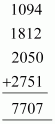∴ Total tickets sold = 7,707

Q.2. Shekhar is a famous cricket player. He has so far scored 6980 runs in test matches. He wishes to complete 10, 000 runs. How many more runs does he need?

Ans:

Runs scored so far = 6980

Runs Shekhar wants to score = 10,000

More runs required = 10,000 − 6980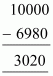∴ Shekhar requires 3,020 more runs.

Q.3. In an election, the successful candidate registered 5, 77, 500 votes and his nearest rival secured 3, 48, 700 votes. By what margin did the successful candidate win the election?

Ans:

Votes secured by successful candidate = 5,77,500

Votes secured by rival = 3,48,700

Margin = 5,77,500 − 3,48,700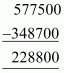∴ Margin = 2,28,800

Q.4. Kirti bookstore sold books worth Rs 2,85,891 in the first week of June and books worth Rs 4,00,768 in the second week of the month. How much was the sale for the two weeks together? In which week was the sale greater and by how much?

Ans:

Value of Books sold in 1st week = Rs 2,85,891

Value of books sold in 2nd week = Rs 4,00,768

Total sale = Sale in 1st week + Sale in 2nd week

= 2,85,891 + 4,00,768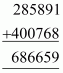The sale for the two weeks together was 6,86,659.

Since 4,00,768 > 2,85,891, sale in 2nd week was greater than 1st week.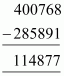∴ The sale in 2nd week was larger than the sale in 1st week by Rs 1,14,877.

Q.5. Find the difference between the greatest and the least number that can be written using the digits 6, 2, 7, 4, 3 each only once.

Ans:

Greatest number = 76432

Smallest number = 23467

Difference = 76432 − 23467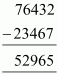Therefore, the difference between the greatest and the least number that can be written using the digits 6, 2, 7, 4, 3 each only once is 52,965.

Q.6. A machine, on an average, manufactures 2,825 screws a day. How many screws did it produce in the month of January 2006?

Ans:

Screws produced in one day = 2,825

Days in January = 31

Screws produced in 31 days = 2825 × 31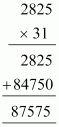Therefore, screws produced during Jan, 06 = 87,575

Q.7. A merchant had Rs 78,592 with her. She placed an order for purchasing 40 radio sets at Rs 1200 each. How much money will remain with her after the purchase?

Ans:

Cost of one radio set = Rs 1200

Cost of 40 radio sets = 1200 × 40 = Rs 48000

Money with Merchant = Rs 78,592

Money spent = Rs 48,000

Money left = 78592 − 48000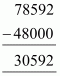Therefore, Rs 30,592 will remain with her after the purchase.

Q.8. A student multiplied 7236 by 65 instead of multiplying by 56. By how much was his answer greater than the correct answer? (Hint: Do you need to do both the multiplications?)

Ans:

Difference between 65 and 56 = 9

Difference in the answer = 7236 × 9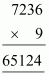Therefore, his answer was greater than the correct answer by 65,124.

Q.9. To stitch a shirt, 2m 15 cm cloth is needed. Out of 40 m cloth, how many shirts can be stitched and how much cloth will remain? (Hint: convert data in cm.)

Ans:

2 m 15 cm = 215 cm (1 m = 100 cm)

40 m = 40 × 100

= 4000 cm

Cloth required for one shirt = 215 cm

Number of shirts that can be stitched out of 4000 cm = 4000 ÷ 215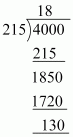Therefore, 18 shirts can be made. 130 cm, i.e. 1 m 30 cm, cloth will remain.

Q.10. Medicine is packed in boxes, each weighing 4 kg 500 g. How many such boxes can be loaded in a van which cannot carry beyond 800 kg?

Ans:

1 kg = 1000 g

4 kg 500 g = 4500 g

800 kg = 800 × 1000 = 800000 g

Number of boxes that can be loaded in the van = 800000 ÷ 4500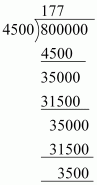Hence, 177 boxes at maximum can be loaded in the van.

Q.11. The distance between the school and the house of a student’s house is 1 km 875 m. Everyday she walks both ways. Find the total distance covered by her in six days.

Ans:

Distance between school and house = 1 km 875 m

Now, 1 km = 1000 m

1 km 875 m = 1875 m

Distance covered each day = 1875 × 2 = 3750 m

Distance covered in 6 days = 3750 × 6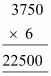Therefore, distance covered in 6 days = 22,500 m

= 22.5 km or 22 km 500 m

Q.12. A vessel has 4 litres and 500 ml of curd. In how many glasses, each of 25 ml capacity, can it be filled?

Ans:

Capacity of vessel = 4 l 500 ml

= 4500 ml (1 l = 1000 ml)

Capacity of a glass = 25 ml

Number of glasses that can be filled = 4500 ÷ 25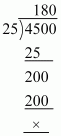∴ 180 glasses can be filled.

Offer running on EduRev: Apply code STAYHOME200 to get INR 200 off on our premium plan EduRev Infinity!

## Mathematics (Maths) Class 6

191 videos|224 docs|43 tests

,

,

,

,

,

,

,

,

,

,

,

,

,

,

,

,

,

,

,

,

,

;